# Train 3-D Speech Enhancement Network Using Deep Learning

In this example, you train a filter and sum network (FaSNet)  to perform speech enhancement (SE) using ambisonic data. The model has been updated to use stacked dual-path recurrent neural networks (DPRNNs) which enable memory-efficient joint modeling of short- and long-term sequences . To explore the model trained in this example, see 3-D Speech Enhancement Using Trained Filter and Sum Network.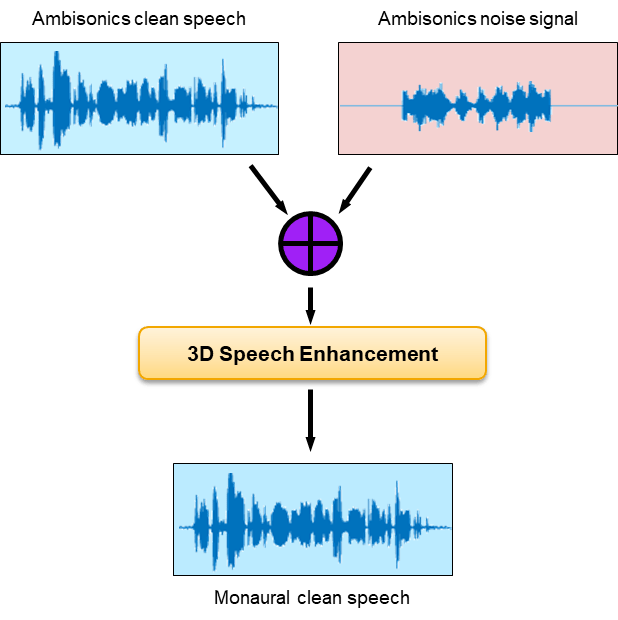### Introduction

The aim of speech enhancement (SE) is to suppress the noise in a noisy speech signal. The SE system may be used as a front end in teleconferencing systems, where intelligibility and listening experience are important metrics, or a speech-to-text system, where the word error rate of the downstream speech-to-text system is the important metric.

In this example, you use the L3DAS 2021 Task 1 dataset  to train and evaluate a model that uses B-format ambisonic data to perform speech enhancement. The enhanced speech is output as a mono audio signal. To explore the model trained in this example, see 3-D Speech Enhancement Using Trained Filter and Sum Network.

#### Optionally Reduce Data Set

To train the network with the entire data set, set `speedupExample` to `false`. To run this example quickly, set `speedupExample` to `true`. This network requires a large amount of data to achieve reasonable results.

`speedupExample =false;`

This example uses the L3DAS21 task 1 challenge data set . The train data sets contains 2 multiple-source and multiple-perspective (MSMP) B-format ambisonic recordings collected at a sampling rate of 16 kHz. The two microphones are labeled as "A" and "B". In this example, you discard recordings from microphone B. Including microphone B data in the training should improve the final performance. The train and validation splits are provided with the data set. The 3-D speech enhancement data set contains more than 30,000 virtual 3-D audio environments with a duration up to 10 seconds. Each sample contains a spoken voice and other office-like background noises. The target data is the clean monophonic voice signal. The `dev` dataset is 2.6 GB, the `train100` dataset is 7.6 GB, and the `train360` dataset is 28.6 GB.

Download the data set and point to it using `audioDatastore`.

```downloadLocation = tempdir; datasetLocationDev = fullfile(downloadLocation,"L3DAS_Task1_dev"); datasetLocationTrain100 = fullfile(downloadLocation,"L3DAS_Task1_train100"); datasetLocationTrain360 = fullfile(downloadLocation,"L3DAS_Task1_train360"); if speedupExample if ~datasetExists(datasetLocationDev) urlDev = "https://zenodo.org/record/4642005/files/L3DAS_Task1_dev.zip"; unzip(urlDev,downloadLocation) end ads = audioDatastore(fullfile(downloadLocation,"L3DAS_Task1_dev"),IncludeSubfolders=true); else if ~datasetExists(datasetLocationDev) urlDev = "https://zenodo.org/record/4642005/files/L3DAS_Task1_dev.zip"; unzip(urlDev,downloadLocation) end if ~datasetExists(datasetLocationTrain100) urlTrain100 = "https://zenodo.org/record/4642005/files/L3DAS_Task1_train100.zip"; unzip(urlTrain100,downloadLocation) end if ~datasetExists(datasetLocationTrain360) urlTrain360 = "https://zenodo.org/record/4642005/files/L3DAS_Task1_train360.zip"; unzip(urlTrain360,downloadLocation) end adsValidation = audioDatastore(fullfile(downloadLocation,"L3DAS_Task1_dev"),IncludeSubfolders=true); adsTrain = audioDatastore([fullfile(downloadLocation,"L3DAS_Task1_train100"), ... fullfile(downloadLocation,"L3DAS_Task1_train360")],IncludeSubfolders=true); end```

To subset the datastores into targets and predictors, use `subset`. Only use microphone A predictors. Using both microphones should increase model performance at the cost of more training time.

```if speedupExample [~,fileNames] = fileparts(ads.Files); targetFiles = ~endsWith(fileNames,["A","B"]); micAFiles = endsWith(fileNames,"A"); T = subset(ads,targetFiles); X = subset(ads,micAFiles); XTrain = subset(X,1:40); TTrain = subset(T,1:40); XValidation = subset(X,41:50); TValidation = subset(T,41:50); else [~,fileNames] = fileparts(adsTrain.Files); targetFiles = ~endsWith(fileNames,["A","B"]); micAFiles = endsWith(fileNames,"A"); TTrain = subset(adsTrain,targetFiles); XTrain = subset(adsTrain,micAFiles); [~,fileNames] = fileparts(adsValidation.Files); targetFiles = ~endsWith(fileNames,["A","B"]); micAFiles = endsWith(fileNames,"A"); TValidation = subset(adsValidation,targetFiles); XValidation = subset(adsValidation,micAFiles); end```

Remove any files that do not overlap between targets and predictors.

```[~,hFiles] = fileparts(TTrain.Files); [~,kFiles] = fileparts(XTrain.Files); kFiles = erase(kFiles,"_A"); validFiles = intersect(kFiles,hFiles); targetValidFiles = ismember(validFiles,kFiles); predictorsValidFiles = ismember(kFiles,validFiles); TTrain = subset(TTrain,targetValidFiles); XTrain = subset(XTrain,predictorsValidFiles); [~,hFiles] = fileparts(TValidation.Files); [~,kFiles] = fileparts(XValidation.Files); kFiles = erase(kFiles,"_A"); validFiles = intersect(kFiles,hFiles); targetValidFiles = ismember(validFiles,kFiles); predictorsValidFiles = ismember(kFiles,validFiles); TValidation = subset(TValidation,targetValidFiles); XValidation = subset(XValidation,predictorsValidFiles);```

To combine the predictor and target datastores so that reading from the combined datastore returns the predictors and associated target, use `combine`.

```dsTrain = combine(XTrain,TTrain); dsValidation = combine(XValidation,TValidation);```

#### Inspect Data

Preview the ambisonic recordings and plot the data.

```predictor = preview(XTrain); target = preview(TTrain); fs = 16e3; % Known sampling rate of data. t = (0:size(target,1)-1)/fs; tiledlayout(2,1,TileSpacing="tight") nexttile plot(t,target) title("Target") xlabel("Time (s)") axis tight nexttile plot(t,predictor) title("Predictor") xlabel("Time (s)") legend(["W","X","Y","Z"]) axis tight```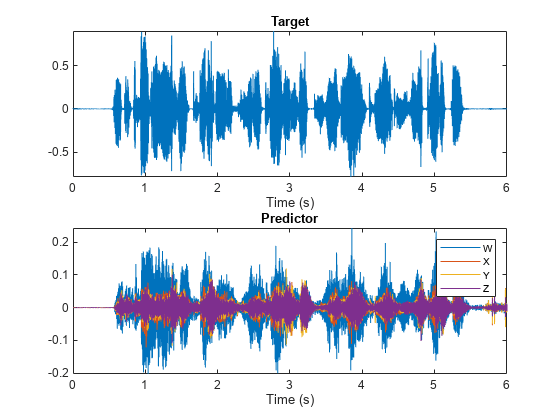Listen to the target data, the mean of the ambisonic channels, or one of the ambisonic channels individually.

```soundSource =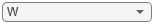predictor(:,1); soundsc(soundSource,fs)```

#### Word Error Rate (WER)

Choosing an appropriate metric to evaluate a SE system performance depends on the final task of the system. For speech-to-text applications, evaluating the word error rate (WER) using the target speech-to-text system is a common approach. For teleconferencing applications, the short-time objective intelligibility measure (STOI) is a common approach. Similarly, the choice of loss function should depend on the final application of the speech enhancement system. In this example, you attempt to optimize the system to reduce WER for a downstream speech-to-text system. One option for the loss function is to use the WER directly, however this can be prohibitively time-consuming for training, and couples the speech enhancement module tightly with the speech-to-text module. Another approach is to use an auditory-based representation of the targets and predictors and calculate the mean square error between them. This example takes the second approach. To get a baseline for performance analysis, calculate the WER of the target (clean) signal, and the noisy signal using a naive approach to SE (mean over channels). The supporting function, `wordErrorRate`, uses the `wav2vec2.0` option of the `speech2text` functionality. If you have not downloaded the pretrained wav2vec 2.0 model, the function throws an error with a link to the download. The WER is calculated using Text Analytics Toolbox™.

```tds = fileDatastore(datasetLocationDev, ... ReadFcn=@(x)string(fileread(x)), ... IncludeSubfolders=true,FileExtensions=".txt"); [~,tdsFiles] = fileparts(tds.Files); [~,TValidationFiles] = fileparts(TValidation.Files); validFiles = ismember(tdsFiles,TValidationFiles); tds = subset(tds,validFiles); dsWER = combine(XValidation,TValidation,tds); WERa = wordErrorRate(dsWER,TargetWER=true,BaselineWER=true);```
```progress = 1.2.3.4.5.6.7.8.9.10.11.12.13.14.15.16.17.18.19.20.21.22.23.24.25.26.27.28.29.30.31.32.33.34.35.36.37.38.39.40.41.42.43.44.45.46.47.48.49.50.51.52.53.54.55.56.57.58.59.60.61.62.63.64.65.66.67.68.69.70.71.72.73.74.75.76.77.78.79.80.81.82.83.84.85.86.87.88.89.90.91.92.93.94.95.96.97.98.99....complete. ```
`WERa.Target`
```ans = 0.0296 ```
`WERa.Baseline`
```ans = 0.4001 ```

### Filter and Sum Network (FaSNet)

This example uses the filter and sum network (FaSNet) architecture with dual-path recurrent neural networks (DPRNN). FaSNet is a time-domain adaptive beamforming framework consisting of two stages:

1. Estimate the beamforming filter for selected reference channel, and then denoise the reference signal.

2. Beamform remaining channels using the denoised reference channel.

The FaSNet using DPRNN architecture is implemented in the supporting function `FaSNet`, which is in the current folder when you open this example.

#### Stage 1: Denoise Reference Mic

In stage one, a normalized cross correlation (NCC) metric is computed between the windows of the reference channel with context and windows of the remaining channels. This example uses cosine similarity as the correlation metric. The metric is pooled across the channels, passed through a temporal convolutional network (TCN), and then through the beamforming filter learner. The output from the beamformer module blocks is then used to filter the reference channel.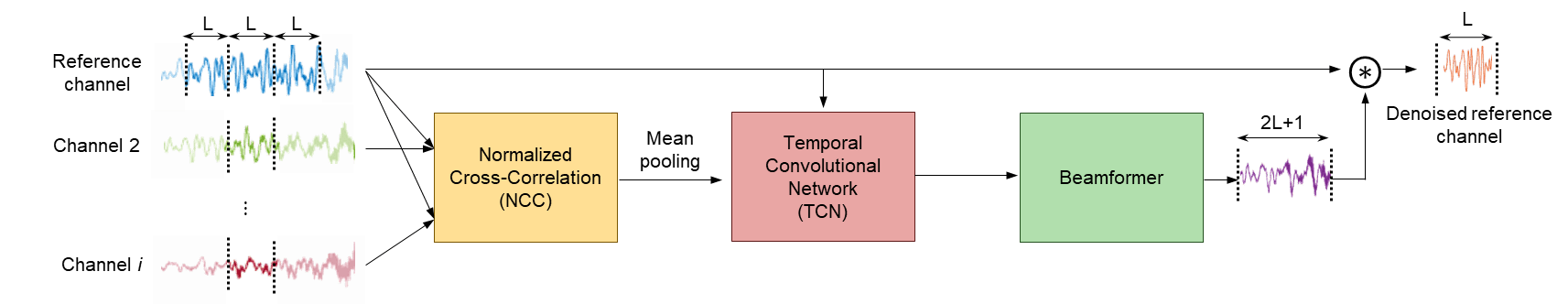#### Stage 2: Create Beamformed Signal

In stage two, a NCC metric is computed between the denoised windows of the reference channel and windows of the remaining channels with context. A beamforming filter is learned for each of the remaining channels. Each channel is separately denoised, and then the channels are summed to create the beamformed final signal.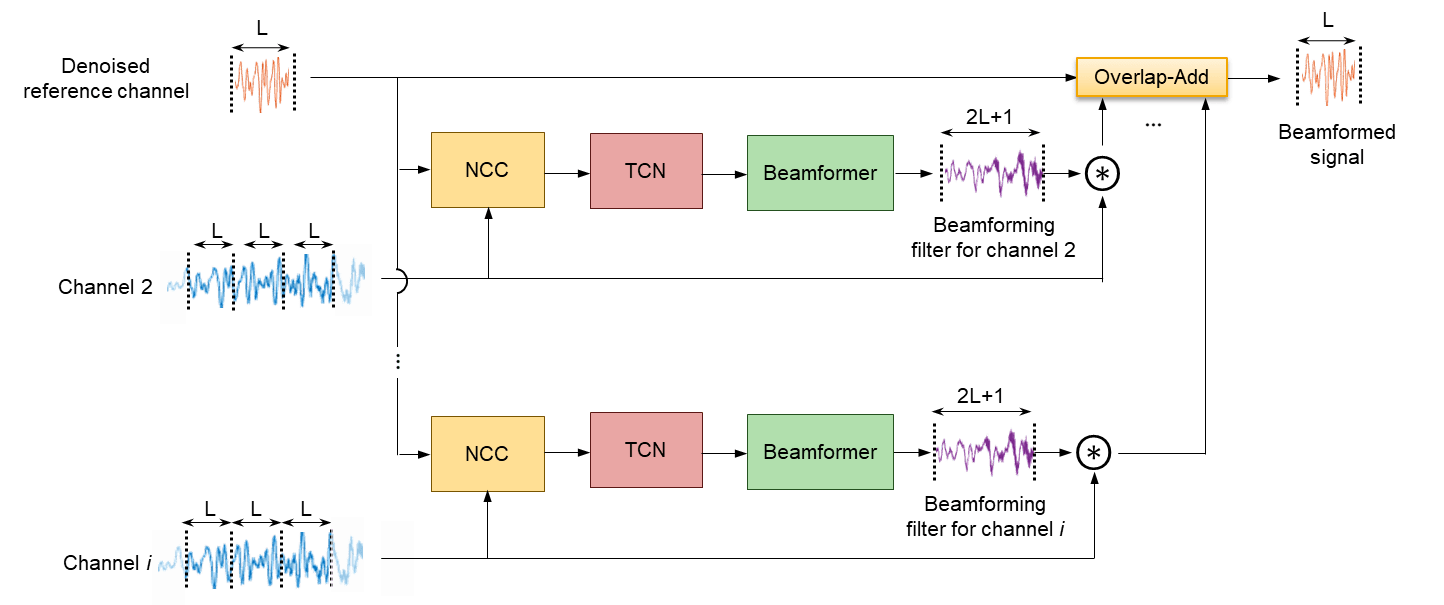#### Beamformer

The beamformer module follows the design of  except replaces the stacked TCN blocks with stacked DPRNN blocks.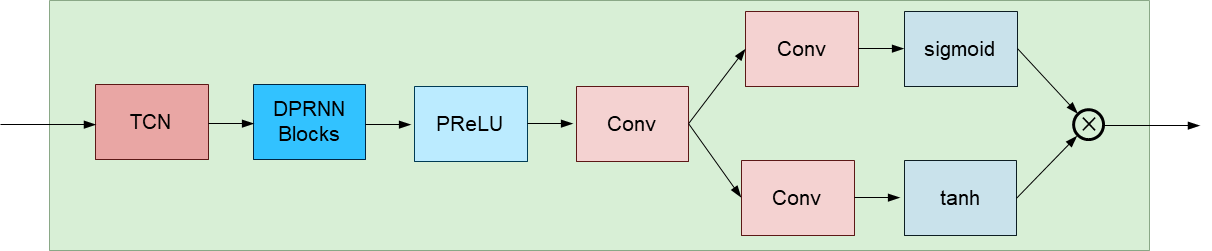#### Dual-Path Recurrent Neural Network

Dual-path recurrent neural networks (DPRNN) were introduced in  as a method of organizing RNN layers in a deep structure to model extremely long sequences. DPRNN splits sequential input into chunks and then applies intra- and inter-chunk operations iteratively. The approach has been shown to perform as well or better than 1-D CNN architectures with a significantly smaller model size. The DPRNN model consists of three stages: segmentation, DPRNN blocks (which may be stacked), and then overlap-add reconstruction.

Segmentation

The sequence is split into `S` segments of length `K` with overlap `P`. In this example, `K = 2P`.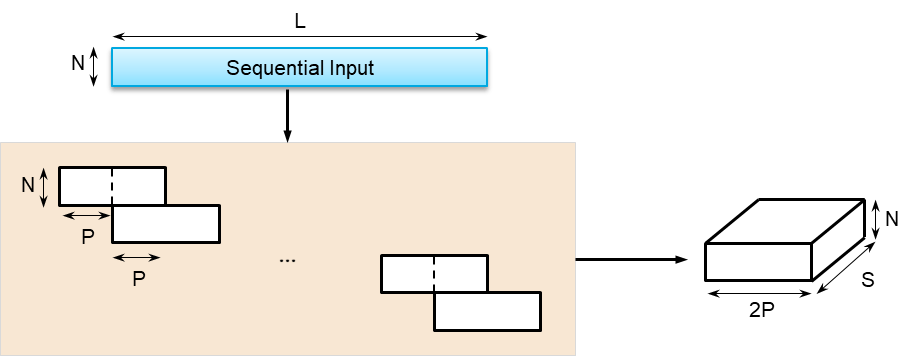DPRNN Block

The segmented signal passes through `B` DPRNN blocks. In this example, `B` is set to `6`. Each block contains two sub-modules corresponding to intra- and inter-chunk processing. The intra-chunk RNN is always bi-directional. The intra-chunk RNN processes each segment individually. The inter-chunk RNN may be uni- or bi-directional, depending on latency requirements of your system. In this example, the inter-chunk RNN is bi-directional. The inter-chunk RNN processes along the stacked dimension of length `S`. The output of each DPRNN block is the same size as the input.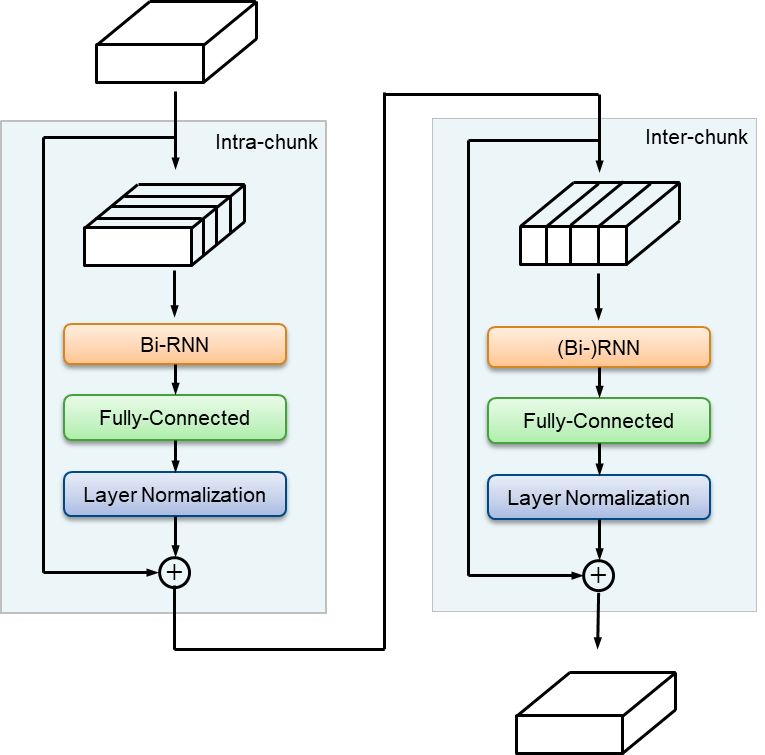The output from the stacked DPRNN blocks is overlapped and added to reconstruct the sequence data.#### Define Parameters

Define system-level, FaSNet-level, and DPRNN-level parameters.

```% System-level parameters parameters.SampleRate = fs; parameters.AnalysisLength = 2*parameters.SampleRate; % FaSNet-level parameters parameters.WindowLength = 256; % L in FaSNet parameters.EncoderDimension = 64; % Number filters in TCN parameters.NumDPRNNBlocks = 6; % Number of stacked DPRNN blocks % DPRNN-level parameters parameters.FeatureDimension = 64; % Number of filters in convolutional blocks parameters.SegmentSize = 24; % 2P parameters.HiddenDimension = 128; % RNN size```

#### Initialize Network Learnables

Use the supporting function, `intitializeLearnables`, to initialize the FaSNet architecture for the specified parameters.

`learnables = initializeLearnables(parameters);`

### Input Pipeline

Define the mini-batch size. Create `minibatchqueue` (Deep Learning Toolbox) objects to read mini-batches from the training data set. The supporting function `preprocessMiniBatch` randomly selects a single clip of the specified `parameters.AnalysisLength` from each audio file in the mini-batch. This approach avoids the need to buffer and save individual audio files, which reduces disk space requirements. The approach has the added benefit of changing the exact sequences seen between epochs. However, this approach puts more emphasis on shorter files in the training data.

```miniBatchSize =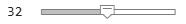32; mbqTrain = minibatchqueue(dsTrain, ... MiniBatchSize=miniBatchSize, ... MiniBatchFcn=@(x,t)preprocessMiniBatch(x,t,parameters.AnalysisLength), ... DispatchInBackground=canUseParallelPool); mbqValidation = minibatchqueue(dsValidation, ... MiniBatchSize=miniBatchSize, ... MiniBatchFcn=@(x,t)preprocessMiniBatch(x,t,parameters.AnalysisLength), ... DispatchInBackground=canUseParallelPool);```

### Training Options

Choose a loss metric as `auditory-mse`, `sample-mse`, or `sample-sisdr`.

• `auditory-mse`: Use the mean-square-error (MSE) between a mel spectrogram computed from the target and a mel spectrogram computed from the prediction.

• `sample-mse`: Use the sample-level MSE between the target and predictor.

• `sample-sisdr`: Use the sample-level scale-invariant signal-to-distortion ratio defined in .

`lossType =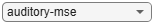"auditory-mse";`

Define the maximum number of epochs, the initial learn rate, and piece-wise learning parameters such as validation patience, learn rate drop factor, and minimum learn rate. The default settings correspond to those reported in  for the task of speaker separation.

```maxEpochs =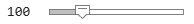100; initialLearnRate =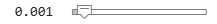0.001; validationPatience =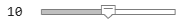10; learnRateDropFactor =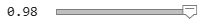0.98; learnRateDropPeriod =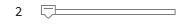2; if speedupExample maxEpochs = 1; end```

Initialize parameters required for the training loop.

```iteration = 0; bestLoss = inf; averageGrad = []; averageSqGrad = []; learnRate = initialLearnRate;```

### Train Network

Create a `trainingProgressMonitor` to monitor the training loss and validation loss while training.

```monitor = trainingProgressMonitor( ... Metrics=["TrainingLoss","ValidationLoss"], ... Info=["Epoch","LearnRate"]); groupSubPlot(monitor,"Loss",["TrainingLoss","ValidationLoss"])```

Record the loss for the untrained network.

```validationLoss = mbqLoss(mbqValidation,learnables,parameters,lossType); recordMetrics(monitor,0,ValidationLoss=validationLoss)```

Run the training loop.

```for epoch = 1:maxEpochs % Update plot info updateInfo(monitor,Epoch=epoch,LearnRate=learnRate) % Shuffle dataset each epoch shuffle(mbqTrain) while hasdata(mbqTrain) iteration = iteration + 1; % Get next mini batch [X,T] = next(mbqTrain); % Pass the predictors through the network and return the loss and % gradients. [loss,gradients] = dlfeval(@modelLoss,learnables,parameters,X,T,lossType); % Update the network parameters using the ADAM optimizer. [learnables,averageGrad,averageSqGrad] = adamupdate(learnables,gradients, ... averageGrad,averageSqGrad,iteration,learnRate); % Update training progress visualization loss = gather(extractdata(loss)); recordMetrics(monitor,iteration,TrainingLoss=loss) if monitor.Stop break end end if monitor.Stop break end % Compute validation loss validationLoss = mbqLoss(mbqValidation,learnables,parameters,lossType); % Update validation progress visualization recordMetrics(monitor,iteration,ValidationLoss=validationLoss) % Checkpoint if validationLoss < bestLoss bestLoss = validationLoss; bestLossEpoch = epoch; save("CheckPoint.mat","bestLoss","learnables","epoch", ... "averageGrad","averageSqGrad","iteration","learnRate") end if (epoch - bestLossEpoch) > validationPatience display("Validation loss did not improve for "+validationPatience+" epochs.") break end % Reduce the learning rate according to schedule if rem(epoch,learnRateDropPeriod)==0 learnRate = learnRate*learnRateDropFactor; end end```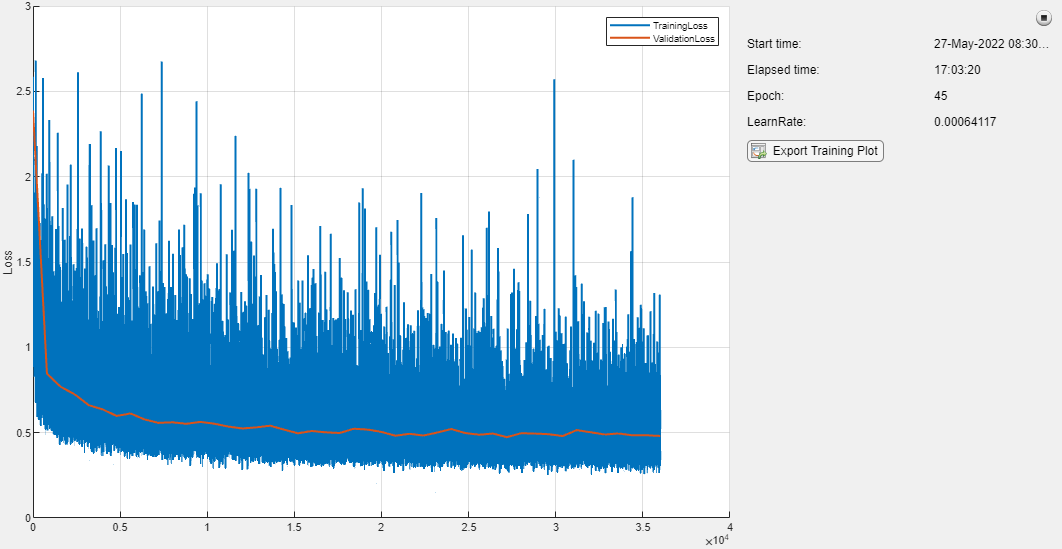``` "Validation loss did not improve for 10 epochs." ```

### Evaluate System

`load("CheckPoint.mat")`

#### Spot Check Performance

Compare the results of the baseline speech enhancement approach against the FaSNet approach using listening tests and common metrics.

```dsValidation = shuffle(dsValidation); [x,t] = read(dsValidation); predictor = x{1}; target = x{2};```

As a baseline speech enhancement system, simply take the mean of the predictors across the channels.

`yBaseline = mean(predictor,2);`

Pass the noisy speech through the network. The network was trained to process data in 2-second segments. The architecture does accept longer and shorter segments, but performs best on inputs of the same size as it was trained on. Use the `preprocessSignal` supporting function to split the audio input into the same segment length as your model was trained on. Pass the segments through the FaSNet model. Treat each segment individually by placing the segment dimension along the third dimension, which the FaSNet model recognizes as the batch dimension.

```y = preprocessSignal(predictor,parameters.AnalysisLength); y = FaSNet(dlarray(y),parameters,learnables); y = gather(extractdata(y)); % Convert to regular array y = y(:); % Concatenate the segments y = y(1:size(predictor,1)); % Trim off any zero-padding used to make complete segments```

Listen to the clean, baseline speech enhanced, and FaSNet speech enhanced signals.

```dur = size(target,1)/fs; soundsc(target,fs),pause(dur+1) soundsc(yBaseline,fs),pause(dur+1) soundsc(y,fs),pause(dur+1)```

Compute the baseline and FaSNet sample MSE, auditory-based MSE, and SISDR. Another common metric not implemented in this example is short-time objective intelligibility (STOI) , which is often used both as a training loss function and for system evaluation.

```yBaselineMSE = 2*mse(yBaseline,target,DataFormat="TB")/size(target,1); yMSE = 2*mse(y,target,DataFormat="TB")/size(target,1); yABaseline = extractdata(dlmelspectrogram(yBaseline,parameters.SampleRate)); yA = extractdata(dlmelspectrogram(y,parameters.SampleRate)); targetA = extractdata(dlmelspectrogram(target,parameters.SampleRate)); yBaselineAMSE = mse(yABaseline,targetA,DataFormat="CTB")/(size(targetA,1)*size(targetA,2)); yAMSE = mse(yA,targetA,DataFormat="CTB")/(size(targetA,1)*size(targetA,2)); yBaselineSISDR = sisdr(yBaseline,target); ySISDR = sisdr(y,target);```

Plot the target signal, the baseline SE result, and the FaSNet SE result. Display performance metrics in the plot titles.

```tiledlayout(3,1) nexttile plot(yBaseline) title("Baseline:"+" MSE="+yBaselineMSE+" Auditory MSE="+yBaselineAMSE+" SISDR="+yBaselineSISDR) grid on axis tight nexttile plot(y) title("FaSNet: "+" MSE="+yMSE+" Auditory MSE="+yAMSE+" SISDR="+ySISDR) grid on axis tight nexttile plot(target) grid on title("Target") axis tight```#### Word Error Rate

Evaluate the word error rate after FaSNet processing and compare to the target (clean) signal and the baseline approach.

`WER = wordErrorRate(dsWER,parameters,learnables,FaSNetWER=true);`
```progress = 1.2.3.4.5.6.7.8.9.10.11.12.13.14.15.16.17.18.19.20.21.22.23.24.25.26.27.28.29.30.31.32.33.34.35.36.37.38.39.40.41.42.43.44.45.46.47.48.49.50.51.52.53.54.55.56.57.58.59.60.61.62.63.64.65.66.67.68.69.70.71.72.73.74.75.76.77.78.79.80.81.82.83.84.85.86.87.88.89.90.91.92.93.94.95.96.97.98.99....complete. ```
`WERa.Baseline`
```ans = 0.4001 ```
`WER.FaSNet`
```ans = 0.2760 ```
`WERa.Target`
```ans = 0.0296 ```

### References

 Luo, Yi, Cong Han, Nima Mesgarani, Enea Ceolini, and Shih-Chii Liu. "FaSNet: Low-Latency Adaptive Beamforming for Multi-Microphone Audio Processing." In 2019 IEEE Automatic Speech Recognition and Understanding Workshop (ASRU), 260–67. SG, Singapore: IEEE, 2019. https://doi.org/10.1109/ASRU46091.2019.9003849.

 Guizzo, Eric, Riccardo F. Gramaccioni, Saeid Jamili, Christian Marinoni, Edoardo Massaro, Claudia Medaglia, Giuseppe Nachira, et al. "L3DAS21 Challenge: Machine Learning for 3D Audio Signal Processing." In 2021 IEEE 31st International Workshop on Machine Learning for Signal Processing (MLSP), 1–6. Gold Coast, Australia: IEEE, 2021. https://doi.org/10.1109/MLSP52302.2021.9596248.

 Roux, Jonathan Le, et al. "SDR – Half-Baked or Well Done?" ICASSP 2019 - 2019 IEEE International Conference on Acoustics, Speech and Signal Processing (ICASSP), IEEE, 2019, pp. 626–30. DOI.org (Crossref), https://doi.org/10.1109/ICASSP.2019.8683855.

 Luo, Yi, et al. "Dual-Path RNN: Efficient Long Sequence Modeling for Time-Domain Single-Channel Speech Separation." ICASSP 2020 - 2020 IEEE International Conference on Acoustics, Speech and Signal Processing (ICASSP), IEEE, 2020, pp. 46–50. DOI.org (Crossref), https://doi.org/10.1109/ICASSP40776.2020.9054266.

 Taal, Cees H., Richard C. Hendriks, Richard Heusdens, and Jesper Jensen. "An Algorithm for Intelligibility Prediction of Time–Frequency Weighted Noisy Speech." IEEE Transactions on Audio, Speech, and Language Processing 19, no. 7 (September 2011): 2125–36. https://doi.org/10.1109/TASL.2011.2114881.

### Supporting Functions

#### Word Error Rate (WER)

```function out = wordErrorRate(ds,parameters,learnables,nvargs) %wordErrorRate Word error rate (WER) % wordErrorRate(ds,parameters,learnables) calculates the word error rate % over all files in the datastore. Specify ds as a combined datastore that % outputs the predictors and targets and also the text labels. % % wordErrorRate(ds,net,TargetWER=TF1,BaselineWER=TF2,FaSNetWER=TF2) % specifies which signals to calculate the word error rate for. Choose any % combination of target (the clean monoaural signal), baseline (the noisy % ambisonic signal converted to monoaural through channel mean) and FaSNet % (the beamform output from the FaSNet model). By default, WER is computed % for all options. % % This function requires Text Analytics Toolbox(TM). arguments ds parameters = []; learnables = []; nvargs.TargetWER = false; nvargs.BaselineWER = false; nvargs.FaSNetWER = false; nvargs.Verbose = true; end % Create a speech client object to perform transcription. transcriber = speechClient("wav2vec2.0",Segmentation="none"); % Initialize counters editDistanceTotal_t = 0; editDistanceTotal_b = 0; editDistanceTotal_y = 0; numWordsTotal = 0; p = 0; % Reset the datastore reset(ds) fprintf("progress = ") while hasdata(ds) % Read from datastore and unpack. [data,audioInfo] = read(ds); predictors = data{1}; targets = data{2}; txt = lower(data{3}); fs = audioInfo{1}.SampleRate; % Put data on GPU if available if canUseGPU && nvargs.TargetWER targets = gpuArray(targets); end if canUseGPU && (nvargs.BaselineWER || nvargs.FaSNetWER) predictors = gpuArray(predictors); end % Update the total number of words. numWordsTotal = numWordsTotal + numel(split(txt)); % Tokenize the text. tokenizedGroundTruth = tokenizedDocument(txt); tokenizedGroundTruth = correctSpelling(tokenizedGroundTruth); % Update the total edit distance by passing the signal through % speech-to-text, tokenizing the document, and then computing the edit % distance against the ground truth text. if nvargs.TargetWER targetsText = speech2text(transcriber,targets,fs); T = tokenizedDocument(targetsText); T = correctSpelling(T); editDistanceTotal_t = editDistanceTotal_t + editDistance(T,tokenizedGroundTruth); end if nvargs.BaselineWER predictorsTextBaseline = speech2text(transcriber,mean(predictors,2),fs); B = tokenizedDocument(predictorsTextBaseline); B = correctSpelling(B); editDistanceTotal_b = editDistanceTotal_b + editDistance(B,tokenizedGroundTruth); end if nvargs.FaSNetWER x = preprocessSignal(predictors,parameters.AnalysisLength); y = FaSNet(dlarray(x),parameters,learnables); y = y.extractdata(); y = y(:); predictorsText = speech2text(transcriber,y,fs); Y = tokenizedDocument(predictorsText); Y = correctSpelling(Y); editDistanceTotal_y = editDistanceTotal_y + editDistance(Y,tokenizedGroundTruth); end % Print status if nvargs.Verbose && (100*progress(ds))>p+1 p = round(100*progress(ds)); fprintf(string(p)+".") end end fprintf("...complete.\n") % Output the results as a struct. out = struct(); if nvargs.FaSNetWER out.FaSNet = editDistanceTotal_y/numWordsTotal; end if nvargs.BaselineWER out.Baseline = editDistanceTotal_b/numWordsTotal; end if nvargs.TargetWER out.Target = editDistanceTotal_t/numWordsTotal; end end ```

#### Model Loss

```function [loss,gradients] = modelLoss(learnables,parameters,X,T,lossType) %modelLoss Model loss for FaSNet % loss = modelLoss(learnables,parameters,X,T,lossType) calculates the % FaSNet model loss using the specified loss type. Specify learnables and % parameters as the learnables and parameters of the FaSNet model. X and T % are the predictors and targets, respectively. lossType is "sample-mse", % "sample-sisdr", or "auditory-mse". % % [loss,gradients] = modelLoss(...) also calculates the gradients when % training a model. % Beamform ambisonic data using FaSNet Y = FaSNet(X,parameters,learnables); % Compute specified loss type switch lossType case "sample-sisdr" loss = -sisdr(Y,T); loss = sum(loss)/size(T,2); case "sample-mse" loss = 2*mse(Y,T,DataFormat="TB")/size(T,1); case "auditory-mse" Ym = dlmelspectrogram(Y,parameters.SampleRate); Tm = dlmelspectrogram(T,parameters.SampleRate); loss = mse(Ym,Tm,DataFormat="CTB")./(size(Tm,1)*size(Tm,2)); end % If gradients requested, compute them if nargout==2 gradients = dlgradient(loss,learnables); end end```

#### Preprocess Mini Batch

```function [X,T] = preprocessMiniBatch(Xcell,Tcell,N) %preprocessMiniBatch Preprocess mini batch % [X,T] = preprocessMiniBatch(Xcell,Tcell,N) takes the mini-batch of data % read from the combined datastore and preprocesses the data using the % preprocessSignalTrain supporting function. for ii = 1:numel(Xcell) [Xcell{ii},idx] = preprocessSignalTrain(Xcell{ii},Samples=N); Tcell{ii} = preprocessSignalTrain(Tcell{ii},Samples=N,Index=idx); end X = cat(3,Xcell{:}); T = cat(2,Tcell{:}); end```

#### Preprocess Signal for FaSNet

```function y = preprocessSignal(x,L) %preprocessSignal Preprocess signal for FaSNet % y = preprocessSignal(x,L) splits the multi-channel % signal x into analysis frames of length L and hop L. The output is a % L-by-size(x,2)-by-numHop array, where the number of hops depends on the % input signal length and L. % Cast the input to single precision x = single(x); % Get the input dimensions N = size(x,1); nchan = size(x,2); % Pad as necessary. if N<L numToPad = L-N; x = cat(1,x,zeros(numToPad,size(x,2),like=x)); else numHops = floor((N-L)/L) + 1; numSamplesUsed = L+(L*(numHops-1)); if numSamplesUsed < N numSamplesUnused = N-numSamplesUsed; numToPad = L - numSamplesUnused; x = cat(1,x,zeros(numToPad,nchan,like=x)); end end % Buffer the input signal x = audio.internal.buffer(x,L,L); % Reshape the signal to Time-Channel-Hop. numHops = size(x,2)/nchan; x = reshape(x,L,numHops,nchan); y = permute(x,[1,3,2]); end```

#### Mel Spectrogram Compatible with `dlarray`

```function y = dlmelspectrogram(x,fs) %dlmelspectrogram Mel spectrogram compatible with dlarray % y = dlmelspectrogram(x,fs) computes a mel spectrogram from the audio % input. persistent win overlap fftLength filterBank if isempty(filterBank) win = hann(round(0.03*fs),"periodic"); overlap = round(0.02*fs); fftLength = numel(win); filterBank = designAuditoryFilterBank(fs,FFTLength=fftLength); end % Short-time Fourier transform [yr,yi] = dlstft(x,DataFormat="TBC", ... Window=win,OverlapLength=overlap,FFTLength=fftLength); % Power spectrum y = abs(yr).^2 + abs(yi).^2; % Apply filter bank y = permute(y,[1,4,3,2]); % FFTLength-by-NumHops-by-BatchSize y = pagemtimes(filterBank,y); % NumBins-by-NumHops-by-BatchSize % Apply log10. y = log(y+eps)/log(10); end```

#### Scale-Invariant Signal-to-Distortion Ratio (SDR)

```function metric = sisdr(y,t) %sisdr Scale-Invariant Signal-to-Distortion Ratio (SDR) % metric = sisdr(estimate,target) calculates the scale-invariant SDR % described in . % %  Roux, Jonathan Le, et al. "SDR – Half-Baked or Well Done?" ICASSP 2019 - % 2019 IEEE International Conference on Acoustics, Speech and Signal % Processing (ICASSP), IEEE, 2019, pp. 626–30. DOI.org (Crossref), % https://doi.org/10.1109/ICASSP.2019.8683855. y = y - mean(y,1); t = t - mean(t,1); alpha = sum(y.*t,1)./(sum(t.^2,1) + eps); etarget = alpha.*t; eres = y - etarget; top = sum(etarget.^2); bottom = sum(eres.^2); metric = 10*log(top./(bottom+eps))/log(10); end```

#### Preprocess Signal for Training

```function [y,idx] = preprocessSignalTrain(x,options) %preprocessSignalTrain Preprocess signal for training % y = preprocessSignalTrain(x) clips out 32000 contiguous samples from x % and returns as y. The clip starting point is determined randomly. If x is % less than 32000, the signal is padded to 32000. % % y = preprocessSignalTrain(x,Samples=N) specifies the number of samples to % clip as N. If unspecified, Samples defaults to 32000. % % y = preprocessSignalTrain(...,Index=K) specifies the starting index for % clipping. If unspecified, Index is selected randomly with the condition % that there are N samples in the clip. arguments x options.Samples = 32000 options.Index = [] end numSamples = size(x,1); numChannels = size(x,2); % If signal shorter than requested number of samples, pad it. if numSamples < options.Samples x = cat(1,x,zeros(options.Samples - numSamples,numChannels,like=x)); numSamples = options.Samples; end % Choose a random starting index in the signal, then clip a segment out of % the signal. if isempty(options.Index) idx = randi(numSamples-options.Samples+1); else idx = options.Index; end y = x(idx:idx+options.Samples-1,:); end```

#### Calculate Loss Over Mini-Batch Queue

```function loss = mbqLoss(mbq,learnables,parameters,lossType) %mbqLoss Mini-batch queue loss % loss = mbqLoss(mbq,learnables,parameters) calculates the total loss over % the mini-batch queue. numMiniBatch = 0; validationLoss = 0; reset(mbq) while hasdata(mbq) [X,T] = next(mbq); numMiniBatch = numMiniBatch + 1; validationLoss = validationLoss + modelLoss(learnables,parameters,X,T,lossType); end loss = validationLoss/numMiniBatch; end```

#### Initialize FaSNet Learnables

```function learnables = initializeLearnables(parameters) %initializeLearnables Initialize FaSNet learnables % learnables = initializeLearnables(parameters) creates a structure % containing the randomly initialized learnable weights of FaSNet. validateattributes(parameters.SegmentSize,["single","double"],["even","positive"],"intializeLearnables","SegmentSize") validateattributes(parameters.WindowLength,["single","double"],["even","positive"],"initialzieLearnables","WindowLenth") filterDimension = 2*parameters.WindowLength+1; learnables.TCN.conv.weight = dlarray(permute(initializeGlorot(1,parameters.EncoderDimension,3*parameters.WindowLength),[2,1,3])); learnables.TCN.norm.offset = dlarray(zeros(parameters.EncoderDimension,1,"single")); learnables.TCN.norm.scaleFactor = dlarray(ones(parameters.EncoderDimension,1,"single")); for jj = 1:2 % Loop over reference mic and other mics learnables.("Beamformer"+jj).BN.conv.weight = dlarray(squeeze(initializeGlorot(1,parameters.FeatureDimension,filterDimension + parameters.EncoderDimension))); for ii = 1:parameters.NumDPRNNBlocks % Loop over DPRNN blocks learnables.("Beamformer"+jj).("DPRNN_" + ii).("pass"+1).rnn.forward.weights = dlarray(initializeGlorot(parameters.HiddenDimension*4,parameters.FeatureDimension,1)); learnables.("Beamformer"+jj).("DPRNN_" + ii).("pass"+1).rnn.forward.recurrentWeights = dlarray(initializeOrthogonal(parameters.HiddenDimension)); learnables.("Beamformer"+jj).("DPRNN_" + ii).("pass"+1).rnn.forward.bias = dlarray(permute(initializeUnitForgetGate(parameters.HiddenDimension),[2,1])); learnables.("Beamformer"+jj).("DPRNN_" + ii).("pass"+1).rnn.reverse.weights = dlarray(initializeGlorot(parameters.HiddenDimension*4,parameters.FeatureDimension,1)); learnables.("Beamformer"+jj).("DPRNN_" + ii).("pass"+1).rnn.reverse.recurrentWeights = dlarray(initializeOrthogonal(parameters.HiddenDimension)); learnables.("Beamformer"+jj).("DPRNN_" + ii).("pass"+1).rnn.reverse.bias = dlarray(permute(initializeUnitForgetGate(parameters.HiddenDimension),[2,1])); learnables.("Beamformer"+jj).("DPRNN_" + ii).("pass"+1).projection.weights = dlarray(initializeGlorot(parameters.FeatureDimension,2*parameters.HiddenDimension,1)); learnables.("Beamformer"+jj).("DPRNN_" + ii).("pass"+1).projection.bias = dlarray(initializeZeros([1,parameters.FeatureDimension])); learnables.("Beamformer"+jj).("DPRNN_" + ii).("pass"+1).norm.offset = dlarray(initializeZeros([1,parameters.FeatureDimension])); learnables.("Beamformer"+jj).("DPRNN_" + ii).("pass"+1).norm.scaleFactor = dlarray(initializeOnes([1,parameters.FeatureDimension])); learnables.("Beamformer"+jj).("DPRNN_" + ii).("pass"+2).rnn.weights = dlarray(initializeGlorot(parameters.HiddenDimension*4,parameters.FeatureDimension,1)); learnables.("Beamformer"+jj).("DPRNN_" + ii).("pass"+2).rnn.recurrentWeights = dlarray(initializeOrthogonal(parameters.HiddenDimension)); learnables.("Beamformer"+jj).("DPRNN_" + ii).("pass"+2).rnn.bias = dlarray(permute(initializeUnitForgetGate(parameters.HiddenDimension),[2,1])); learnables.("Beamformer"+jj).("DPRNN_" + ii).("pass"+2).rnn.reverse.weights = dlarray(initializeGlorot(parameters.HiddenDimension*4,parameters.FeatureDimension,1)); learnables.("Beamformer"+jj).("DPRNN_" + ii).("pass"+2).rnn.reverse.recurrentWeights = dlarray(initializeOrthogonal(parameters.HiddenDimension)); learnables.("Beamformer"+jj).("DPRNN_" + ii).("pass"+2).rnn.reverse.bias = dlarray(permute(initializeUnitForgetGate(parameters.HiddenDimension),[2,1])); learnables.("Beamformer"+jj).("DPRNN_" + ii).("pass"+2).projection.weights = dlarray(initializeGlorot(parameters.FeatureDimension,2*parameters.HiddenDimension,1)); learnables.("Beamformer"+jj).("DPRNN_" + ii).("pass"+2).projection.bias = dlarray(initializeZeros([1,parameters.FeatureDimension])); learnables.("Beamformer"+jj).("DPRNN_" + ii).("pass"+2).norm.offset = dlarray(initializeZeros([1,parameters.FeatureDimension])); learnables.("Beamformer"+jj).("DPRNN_" + ii).("pass"+2).norm.scaleFactor = dlarray(initializeOnes([1,parameters.FeatureDimension])); end learnables.("Beamformer"+jj).Output.prelu.alpha = dlarray(0.25); learnables.("Beamformer"+jj).Output.conv.weight = dlarray(initializeGlorot(parameters.FeatureDimension,parameters.FeatureDimension,1)); learnables.("Beamformer"+jj).Output.conv.bias = dlarray(initializeZeros([1,parameters.FeatureDimension])); learnables.("Beamformer"+jj).GenerateFilter.X1.weight = dlarray(permute(initializeGlorot(parameters.FeatureDimension,filterDimension,1),[2,1])); learnables.("Beamformer"+jj).GenerateFilter.X1.bias = dlarray(initializeZeros([1,filterDimension])); learnables.("Beamformer"+jj).GenerateFilter.X2.weight = dlarray(permute(initializeGlorot(parameters.FeatureDimension,filterDimension,1),[2,1])); learnables.("Beamformer"+jj).GenerateFilter.X2.bias = dlarray(initializeZeros([1,filterDimension])); end function weights = initializeGlorot(filterSize,numChannels,numFilters) sz = [filterSize,numChannels,numFilters]; numOut = prod(filterSize)*numFilters; numIn = prod(filterSize)*numFilters; Z = 2*rand(sz,"single") - 1; bound = sqrt(6/(numIn + numOut)); weights = bound*Z; weights = dlarray(weights); end function parameter = initializeOrthogonal(numHiddenUnits) sz = [4*numHiddenUnits,numHiddenUnits]; Z = randn(sz,"single"); [Q,R] = qr(Z,0); D = diag(R); Q = Q * diag(D./abs(D)); parameter = dlarray(Q); end function bias = initializeUnitForgetGate(numHiddenUnits) bias = zeros(4*numHiddenUnits,1,"single"); idx = numHiddenUnits+1:2*numHiddenUnits; bias(idx) = 1; bias = dlarray(bias); end function parameter = initializeZeros(sz) parameter = zeros(sz,"single"); parameter = dlarray(parameter); end function parameter = initializeOnes(sz) parameter = ones(sz,"single"); parameter = dlarray(parameter); end end```